# Birthdate

Jane on birthday brought 30 lollipops and 24 chewing gum for their friends. How many friends has, if everyone receives the same number of lollipops and chewing gums? How much chewing gum and lollipops got any friend?

x1 =  6
y1 =  5
z1 =  4
x2 =  3
y2 =  10
z2 =  8
x3 =  2
y3 =  15
z3 =  12

### Step-by-step explanation:

${y}_{1}=30\mathrm{/}{x}_{1}=30\mathrm{/}6=5$
${z}_{1}=24\mathrm{/}{x}_{1}=24\mathrm{/}6=4$
${x}_{2}=3=3$
${y}_{2}=30\mathrm{/}{x}_{2}=30\mathrm{/}3=10$
${z}_{2}=24\mathrm{/}{x}_{2}=24\mathrm{/}3=8$
${x}_{3}=2=2$
${y}_{3}=30\mathrm{/}{x}_{3}=30\mathrm{/}2=15$
${z}_{3}=24\mathrm{/}{x}_{3}=24\mathrm{/}2=12$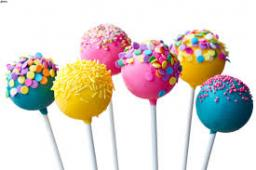Did you find an error or inaccuracy? Feel free to write us. Thank you!Tips to related online calculators
Do you want to calculate greatest common divisor two or more numbers?
Do you want to perform natural numbers division - find the quotient and remainder?

## Related math problems and questions:

• Chewing gums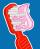For 3 chewing gums, you will pay 20 CZK less than 7 chewing gums. How much is 1 chewing gum and how much does a 5pcs package cost?
• On Children'sOn Children's Day, the organizers bought 252 chewing gums, 396 candies and 108 lollipops. They want to make as many of the same packages as possible. Advise them what to put in each package and how many packages they can make this way.
• Gcd and lcm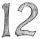Calculate the greatest common divisor and the least common multiple of numbers. a) 16 and 18 b) 24 and 22 c) 45 and 60 d) 36 and 30
• Lcm = 22 + gcdThe least common multiple of two numbers is 22 more than their greatest common divisor. Find these numbers.
• Three friendsThree friends had balls in ratio 2: 7: 4 at the start of the game. Could they have the same number of balls at the end of the game? Write 0, if not, or write the minimum number of balls they had together.
• Ten pupils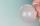10 pupils went to the store. 6 pupils bought lollipops and 9 pupils bought chewing gum. How many pupils have bought both lollipops and chewing gums (if everyone bought something)?
• Mangoes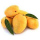Chris has 12 mangoes and Jay has 18 mangoes. Each of them will share the mangoes to their friends . What's the greatest number of mangoes each of their friends get if Chris and Jay will give the same number of mangoes?
• QuotientFind quotient before the bracket - the largest divisor 51 a + 34 b + 68 121y-99z-33
• Common divisorsFind all common divisors of numbers 30 and 45.
• Apples and pearsMom divided 24 apples and 15 pears to children. Each child received the same number of apples and pears - same number as his siblings. How many apples (j=?) and pears (h=?) received each child?
• Florist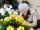Florist has 84 red and 48 white roses. How many same bouquets can he make from them if he must use all roses?
• MO C–I–1 2018An unknown number is divisible by just four numbers from the set {6, 15, 20, 21, 70}. Determine which ones.
• Reminder and quotientThere are given the number C = 281, D = 201. Find the highest natural number S so that the C:S, D:S are with the remainder of 1,
• TissuesThe store got three kinds of tissues - 132 children, 156 women and 204 men. Tissues each species were packed into boxes after the number of pieces the same for all three types (and greatest). Determine the number, if you know that every box has more than
• 6/24 change6/24 change to a fraction in lowest terms
• Trees in alleyThere are four trees in the alley between which the distances are 35m, 15m and 95m. Trees must be laid in the spaces so that the distance is the same and the maximum. How many trees will they put in and what will be the distance between them?
• Identical cubesFrom the smallest number of identical cubes whose edge length is expressed by a natural number, can we build a block with dimensions 12dm x 16dm x 20dm?